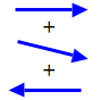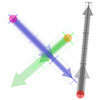#### You may also like### Arrow Arithmetic 1

The first part of an investigation into how to represent numbers using geometric transformations that ultimately leads us to discover numbers not on the number line.### Arrow Arithmetic 2

Introduces the idea of a twizzle to represent number and asks how one can use this representation to add and subtract geometrically.### Arrow Arithmetic 3

How can you use twizzles to multiply and divide?

# Twizzle Arithmetic

##### Age 14 to 16 Challenge Level:

The arithmetic rules for twisted twizzles are the same as the arithmetic rules for untwisted twizzles.

Twisted twizzles are a geometrical picture of complex numbers.

Untwisted twizzles represent the real numbers.

Geometrically, complex arithmetic is a natural extension of real arithmetic because it follows the same rules.